# MapReduce

Problem: Can’t use a single computer to process the data (take too long to process data).

Solution: Use a group of interconnected computers (processor, and memory independent).

Problem: Conventional algorithms are not designed around memory independence.

Solution: MapReduce

Definition. MapReduce is a programming paradigm model of using parallel, distributed algorithims to process or generate data sets. MapRedeuce is composed of two main functions:

Map(k,v): Filters and sorts data.

Reduce(k,v): Aggregates data according to keys (k).

## MapReduce Phases

MapReduce is broken down into several steps:

2. Map
3. Combiner (Optional)
4. Partitioner
5. Shuffle and Sort
6. Reduce
7. Output Format

Record Reader splits input into fixed-size pieces for each mapper.

• The key is positional information (the number of bytes from start of file) and the value is the chunk of data composing a single record.• In hadoop, each map task’s is an input split which is usually simply a HDFS block
• Hadoop tries scheduling map tasks on nodes where that block is stored (data locality)
• If a file is broken mid-record in a block, hadoop requests the additional information from the next block in the series

## Map

Map User defined function outputing intermediate key-value pairskey ($k_{2}$): Later, MapReduce will group and possibly aggregate data according to these keys, choosing the right keys is here is important for a good MapReduce job.

value ($v_{2}$): The data to be grouped according to it’s keys.

## Combiner (Optional)

Combiner UDF that aggregates data according to intermediate keys on a mapper node• This can usually reduce the amount of data to be sent over the network increasing efficiency
• Combiner should be written with the idea that it is executed over most but not all map tasks. ie. $\left(k_{2},v_{2}\right)\mapsto\left(k_{2},v_{2}\right)$

• Usually very similar or the same code as the reduce method.

## Partitioner

Partitioner Sends intermediate key-value pairs (k,v) to reducer by $\mbox{Reducer}=\mbox{hash}\left(\mbox{k}\right)\pmod{R}$• will usually result in a roughly balanced load accross the reducers while ensuring that all key-value pairs are grouped by their key on a single reducer.
• A balancer system is in place for the cases when the key-values are too unevenly distributed.
• In hadoop, the intermediate keys ($k_{2},v_{2}$) are written to the local harddrive and grouped by which reduce they will be sent to and their key.

## Shuffle and Sort

Shuffle and Sort On reducer node, sorts by key to help group equivalent keys## Reduce

Reduce User Defined Function that aggregates data (v) according to keys (k) to send key-value pairs to output## Output Format

Output Format Translates final key-value pairs to file format (tab-seperated by default).### MapReduce Example: Word CountImage Source: Xiaochong Zhang’s Blog

## DAG Models

A more flexible form of MapReduce is used by Spark using Directed Acyclic Graphs (DAG).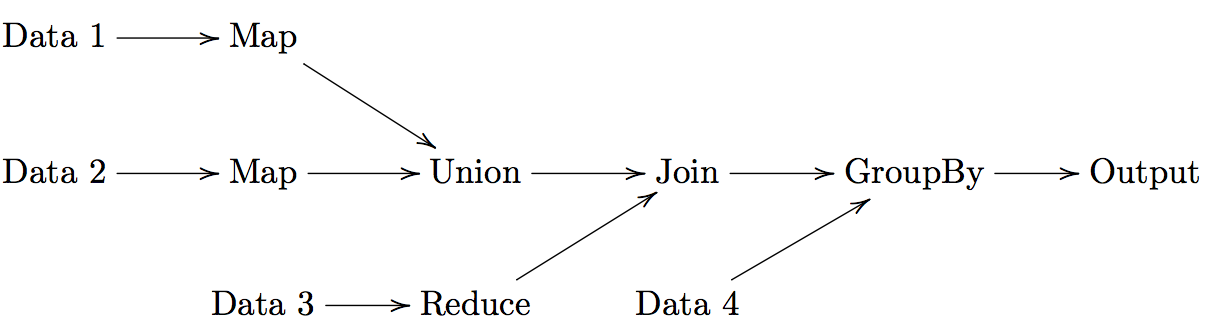For a set of operations:

1. Create a DAG for operations

# MapReduce Programming Models

• Looking for parameter(s) ($\theta$) of a model (mean, parameters of regression, etc.)
1. Partition and Model:
1. Partition data,
2. Apply unbiased estimator,
3. Average results.
2. Sketching / Sufficient Statistics:
1. Partition data,
2. Reduce dimensionality of data applicable to model (sufficient statistic or sketch),
3. Construct model from sufficient statistic / sketch.

## Partition and Model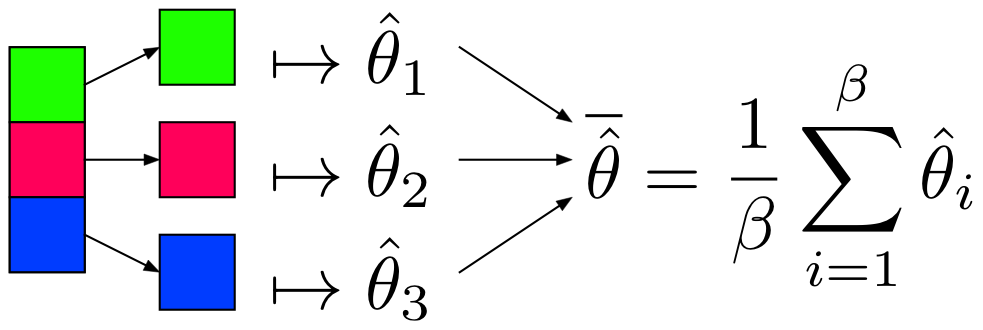### Notes

$\overline{\hat{\theta}}$ is as efficient as $\hat{\theta}$ with the whole dataset when: 1. $\hat{\theta}\sim N\left(\theta,\sigma^{2}\right)$ 2. x is IID and 3. x is equally partitioned

• Can use algorithms already in R, Python to get $\hat{\theta}_i$
• $\overline{\hat{\theta}}\sim N\left(\theta,\frac{\sigma_{\hat{\theta}}^{2}}{\beta}\right)$ by Central Limit Theorem

### Restrictions

• Values must be IID (i.e. not sorted, etc.)
• Model must produce an unbiased estimator of $\theta$, denoted $\hat{\theta}$

## Sketching / Sufficient Statistics

Streaming (Online) Algorithm: An algorithm in which the input is processed item by item. Due to limited memory and processing time, the algorithm produces a summary or “sketch” of the data.

Sufficient Statistic: A statistic with respect to a model and parameter $\theta$, such that no other statistic from the sample will provide additional information.

• Sketching / Sufficient Statistics programming model aims to break down the algorithm into sketches which is then passed off into the next phase of the algorithm.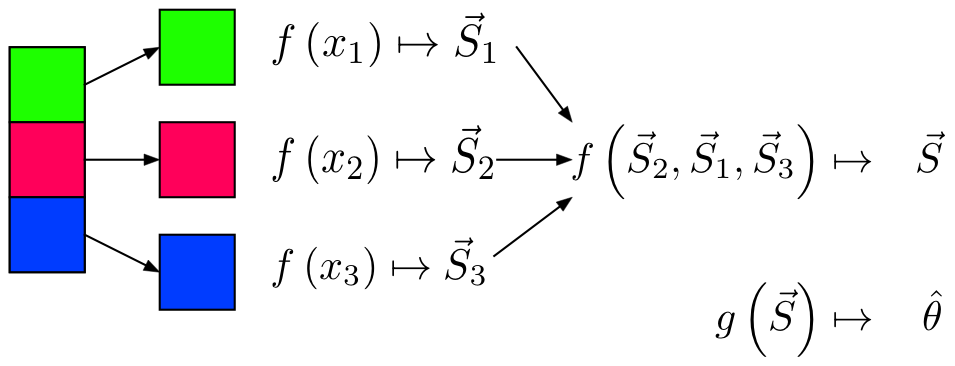### Notes

• Not all algorithms can be broken down this way.

### Restrictions

• All sketches must be communicative and associative
• For each item to be processed, the order of operations must not matter

## Example: Mean

### Partition and Model

%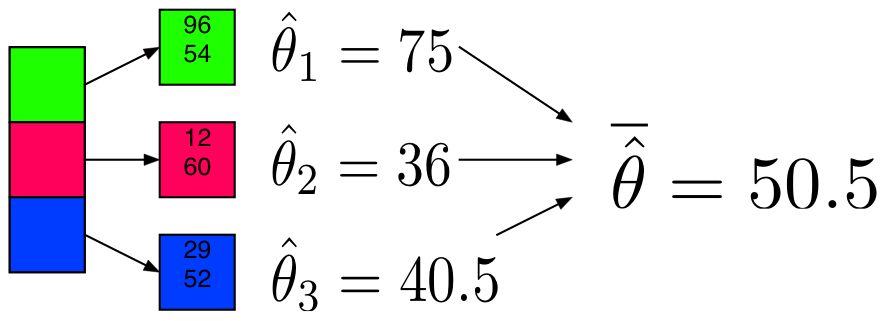### Sufficient Statistic / Sketch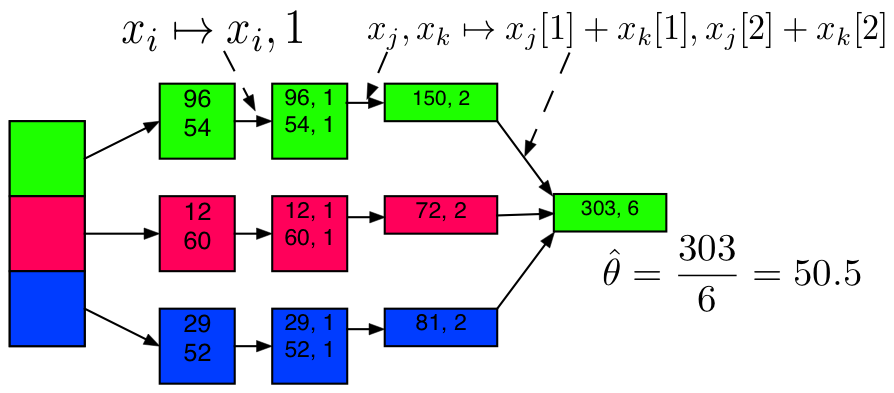## Example: Mean Code

from numpy.random import randint, chisquare
import numpy as np
X = sc.parallelize(randint(0, 101, 200000))
X_part = X.repartition(10).cache()


### Example: Mean + Partition and Model

The partitions are not equally sized, thus we’ll use a weighted average.

%

## Linear Regression

To understand the MapReduce framework, lets solve a familar problem of Linear Regression. For Hadoop/MapReduce to work we MUST figure out how to parallelize our code, in other words how to use the hadoop system to only need to make a subset of our calculations on a subset of our data.

Assumption: The value of p, the number of explanatory variables is small enough for R to easily handle i.e.
$n\gg p$

We know from linear regression, that our estimate of $\hat{\beta}$:
$X^{T}X\hat{\beta}=X^{T}y$

$\left(X^{T}X\right)_{p\times p}$ and $\left(X^{T}y\right)_{p\times1}$ is small enough for R to solve for $\hat{\beta}$, thus we only need $X^{T}X,X^{T}y$ to get $\hat{\beta}$.

To break up this calculation we break our matrix X into submatricies $X_{i}$:
$X=\begin{bmatrix}X_{1}\\ X_{2}\\ X_{3}\\ \vdots\\ X_{n} \end{bmatrix} y=\begin{bmatrix}y_{1}\\ y_{2}\\ y_{3}\\ \vdots\\ y_{n} \end{bmatrix}$

The reason we are returning a list in the map function is because otherwise Reduce will only return a some of the elements of the matricies.

List prevents this by Reduce iterating though the elements of the list (the individual $X_{i}^{T}X_{i}$ matricies) and applying the binary function ‘+’ to each one.

List is used in the reduce function Sum because we will also use this as a combiner function and if we didn’t use a list we would have the same problem as above.

Map Reduce with Examples - February 19, 2015 - Andrew Andrade